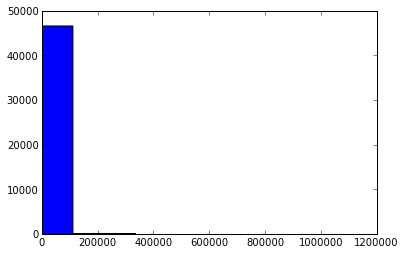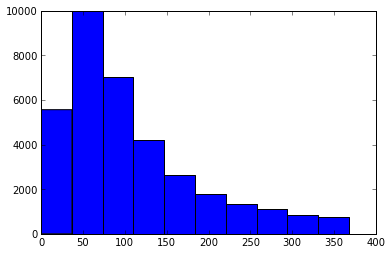How accurate are crowdsourced postcodes?

Posted on 21 Jul 2013

A little while ago at the Cambridge Enterprise Search meetup, Nick Burch of Quanticate shared with us the fantastic story of http://www.npemap.org.uk/. This was setup in the days when the CodePoint dataset, containing the location of all postcodes in the UK, was tightly guarded and ludicrously expensive. It was also I remember a pain to deal with, and still remember the days when the pack of CDs would turn up, and the test server got to spend it's next few days chugging away to rebuild all the indexing.

The NPE project got round the cost element by scanning in all the old, out of copyright, New Popular Edition maps from the '50s and inviting users to pore over and enjoy them, while at the same time tagging areas where they knew the postcode. This provided them with a fantastic data set with a good rough approximation of most of the postcode areas in the UK, that they could then use to provide location and 'nearest to me' type services to various charity clients without breaking the bank on CodePoint.

Nowadays, after much excellent campaigning and the growth of the open data movement, the government has made OS give us all access to the basic version of CodePoint, so this rather fun dataset is no longer really that useful, since we've got the 'real' data. Still, I couldn't help but wonder how they did. How did the crowdsourced version stack up against the correct values?

So, step one, grab the data. The NPE maps site lists all their submitted data and provides it in handy CSV form. It's a bit more of a pain to get the CodePoint data directly (for some unknown reason you have to go through a 'shopping cart' type thing, but at least it's now free). It's also now nicely part of data.gov.

Step 2. Let's have a look. The first major problem is that while the NPE set contains both eastings and northing, and latitude and longitude pairs, the CodePoint set is just eastings and northings. The resolution of both is 1 metre, which is pretty handy.

Here is some python (from an iPython notebook, which by the way are very cool)

In :
import csv
import re
from multiprocessing import Pool
import itertools

pool = Pool(processes=6)

# npe format: <outward>,<inward>,<easting>,<northing>,<WGS84 lat>,<WGS84 long>,<2+6 NGR>,<grid>,<sources>
npe_raw = []

with open('data/npemaps/postcodes.csv', 'rb') as csvfile:
for row in reader:
if not row.startswith('#'):
postcode = row + (' ' if len(row) == 3 else '') + row
npe_raw.append((postcode, int(row), int(row)))
csvfile.close()

print "NPE count {0}".format(len(npe))

npe = sorted(npe_raw, key = lambda x: x)
del npe_raw
NPE count 53109
In :
os_raw = []

with open('data/os/all.csv', 'r') as csvfile:
for row in reader:
os_raw.append((row, int(row), int(row)))
csvfile.close()

os = sorted(os_raw, key = lambda x: x)
del os_raw
In :
import math

def get_distance(a,b):
return math.sqrt(math.pow(a - b,2) + math.pow(a - b,2))

# both inputs are now sorted, go down the list
start = 0
l = len(os)
results = []

with open('distances.csv', 'wb') as csvfile:
csvwriter = csv.writer(csvfile, delimiter=',',
quotechar='"', quoting=csv.QUOTE_MINIMAL)
j=0
for i in npe:
while j < l and os[j] < i:
j+=1
try:
if os[j] == i:
result = i+os[j] + (get_distance(i, os[j]),)
results.append(result)
csvwriter.writerow(result)
except IndexError:
print j, i, l

csvfile.close()
1692241 ('m23 5bt', 383256, 387352) 1692241
In :
import numpy

dists = [row for row in results]

try:
ph = numpy.percentile(dists,75)
mean = numpy.mean(dists)
std = numpy.std(dists)

print "full set mean, std and 75 percentile", mean, std, ph

lower_dists = [row for row in filter(lambda x: x < ph, results)]
print "mean and std below 75 percentile", numpy.mean(lower_dists), numpy.std(lower_dists)
except ValueError:
print "error", len(dists), len(results)
full set mean, std and 75 percentile 3831.69351919 29458.8743255 367.554756011
mean and std below 75 percentile 107.704788278 81.0651732589
In :
import matplotlib.pyplot
%matplotlib inline
matplotlib.pyplot.hist(dists)
Out:
(array([46555,   267,   131,    48,    23,     7,     6,     3,     0,     2]),
array([       0.        ,   111201.87964513,   222403.75929026,
333605.63893539,   444807.51858052,   556009.39822565,
667211.27787078,   778413.15751591,   889615.03716104,
1000816.91680617,  1112018.7964513 ]),
<a list of 10 Patch objects>)Taking out the upper quartile to give more detail to the lower end of the histgram:

In :
matplotlib.pyplot.hist(lower_dists)
Out:
(array([5561, 9980, 7026, 4207, 2651, 1799, 1342, 1099,  861,  755]),
array([   0.        ,   36.74914965,   73.4982993 ,  110.24744895,
146.9965986 ,  183.74574825,  220.4948979 ,  257.24404755,
293.9931972 ,  330.74234685,  367.4914965 ]),
<a list of 10 Patch objects>)So it turns out that the crowdsourced data is actually pretty accurate, give or take a fair number of outliers.

Step 3. Dig into the outliers. Which I may do at another time.

StackOverflow Flair